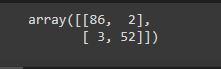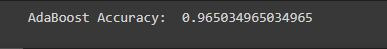# Practical implementation of Adaboost with Python

First of all, we will load all the basic libraries.

import pandas as pd import numpy as np from sklearn.ensemble import AdaBoostClassifier from sklearn.tree import DecisionTreeClassifier from sklearn.datasets import load_breast_cancer from sklearn.model_selection import train_test_split from sklearn.metrics import confusion_matrix, accuracy_score from sklearn.preprocessing import LabelEncoder

Here, I use the breast cancer dataset which can be obtained from sklearn.datasets. It is
also available in Kaggle.

Let’sdeclare our independent variable and the target variable.

X = pd.DataFrame(breast_cancer.data, columns = breast_cancer.feature_names) y = pd.Categorical.from_codes(breast_cancer.target, breast_cancer.target_names)

The target is to classify whether it is a benign or malignant cancer. So, let’s encode the target variable as 0 and 1. 0 for malignant, and 1 for benign.

encoder = LabelEncoder() binary_encoded_y = pd.Series(encoder.fit_transform(y))

Now we split our dataset as train set and test set.

train_X, test_X, train_y, test_y = train_test_split(X, binary_encoded_y, random_state = 1)

we use the Adaboost classifier. Here, we use a decision tree for our model.

classifier = AdaBoostClassifier(DecisionTreeClassifier(max_depth=1), n_estimators=200) classifier.fit(train_X, train_y)

Our Adaboost is fitted now. We will predict the target variable in the test set now.

prediction = classifier.predict(test_X)

Let’s obtain the confusion matrix.

confusion_matrix(test_y, prediction)The main diagonal elements are well-classified data and secondary diagonal elements are misclassified data.

Let’s see the accuracy of classification now.

accuracy = accuracy_score(test_y, prediction) print('AdaBoost Accuracy: ', accuracy)Our accuracy is 96.50%

It is quite a good accuracy.